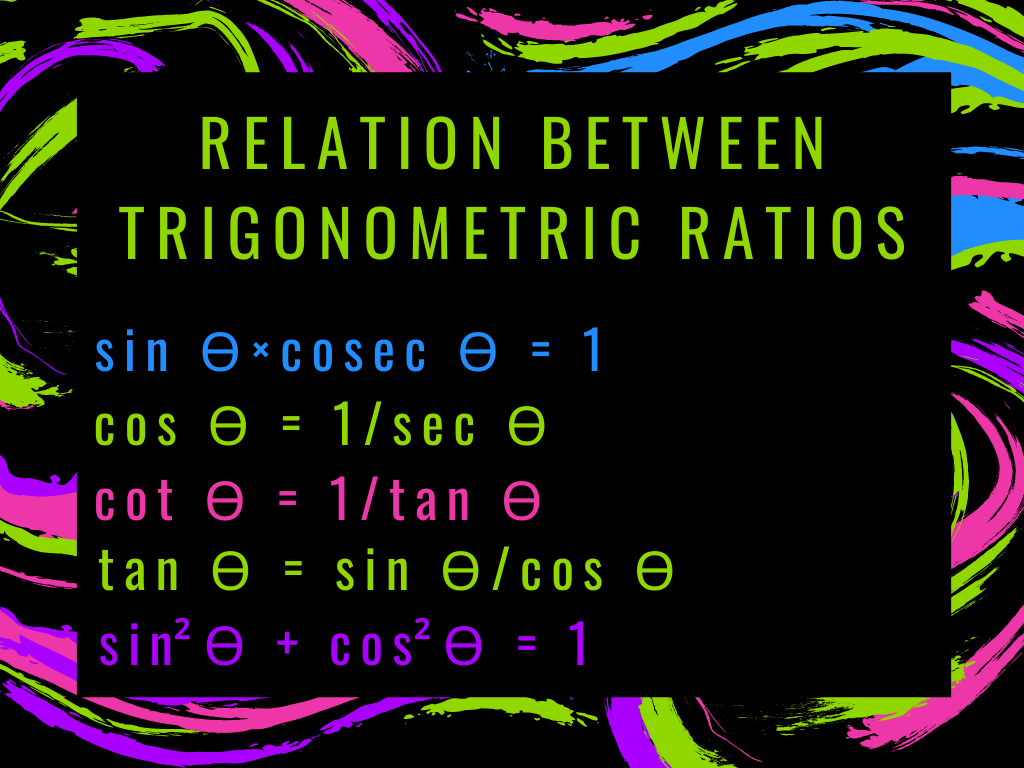## Relation Between Trigonometric Ratios Class 10th

Introduction We know that the reciprocal of sin ϴ, cos ϴ, and tan ϴ is cosec ϴ, sec ϴ, and cot ϴ respectively. Therefore, some formulae are here which show the Relation Between Trigonometric Ratios. Derivation of Formula and Explanation If we assume △PQR,  sin ϴ = PQ/PR    and cosec ϴ = PR/PQ sin ϴ⨯cosec …